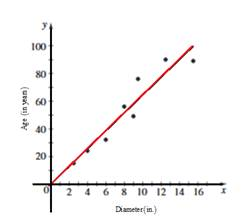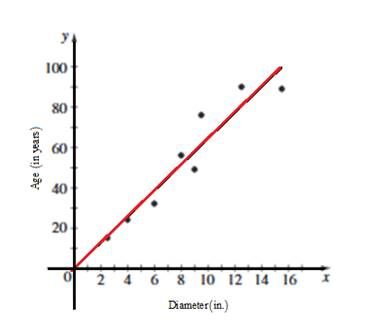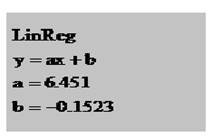# Make a scatter plot of the data.### Precalculus: Mathematics for Calcu...

6th Edition
Stewart + 5 others
Publisher: Cengage Learning
ISBN: 9780840068071### Precalculus: Mathematics for Calcu...

6th Edition
Stewart + 5 others
Publisher: Cengage Learning
ISBN: 9780840068071

#### Solutions

Chapter 1, Problem 3P

a.

To determine

## Make a scatter plot of the data.

Expert Solution### Explanation of Solution

Given information:

To estimate ages of trees, forest rangers use a linear model that relates tree diameter to age. The model is useful because tree diameter is much easier to measure than tree age (which requires special tools for extracting a representative cross-section of the tree and counting the rings). To find the model, use the data in the table, which were collected for a certain variety of oaks.

Calculation:

The scatter plot of the data is given as,Hence, the graph is shown above.

b.

To determine

Expert Solution

y=6.451x0.1523

### Explanation of Solution

Given information:

To estimate ages of trees, forest rangers use a linear model that relates tree diameter to age. The model is useful because tree diameter is much easier to measure than tree age (which requires special tools for extracting a representative cross-section of the tree and counting the rings). To find the model, use the data in the table, which were collected for a certain variety of oaks.

Calculation:

Using a calculator, we find the following regression line that models the data in the form of,

y=ax+b

Using regression calculator,Substituting a=6.451 and b=0.1523 , we get,

y=6.451x0.1523

Hence, the linear function that models the data is y=6.451x0.1523 .

c.

To determine

Expert Solution

116 years.

### Explanation of Solution

Given information:

To estimate ages of trees, forest rangers use a linear model that relates tree diameter to age. The model is useful because tree diameter is much easier to measure than tree age (which requires special tools for extracting a representative cross-section of the tree and counting the rings). To find the model, use the data in the table, which were collected for a certain variety of oaks.

Calculation:

The diameter 18in. corresponds x=18 to in our model. The model gives,

y=6.451(18)0.1523y=116

Hence, the estimated age of an oak whose diameter is 18in. is 116 years.

### Have a homework question?

Subscribe to bartleby learn! Ask subject matter experts 30 homework questions each month. Plus, you’ll have access to millions of step-by-step textbook answers!